Analytics

# Introduction to DAX Variables in Power BIHave you ever had a scenario where you need to use part of your calculation multiple times? You might go and create a table or column for that and then re-use it. However, there are times that you just need that calculation to be re-used multiple times within one place. DAX variables can help you with that. DAX variables are also helpful to make the performance of your calculation better. In this blog, I will explain what DAX variables are, the scenarios for using them, and how they can be better for your Power BI calculations.

## Re-Using Part of the Code

It sometimes happens that you need to re-use part of the code. Consider the example below: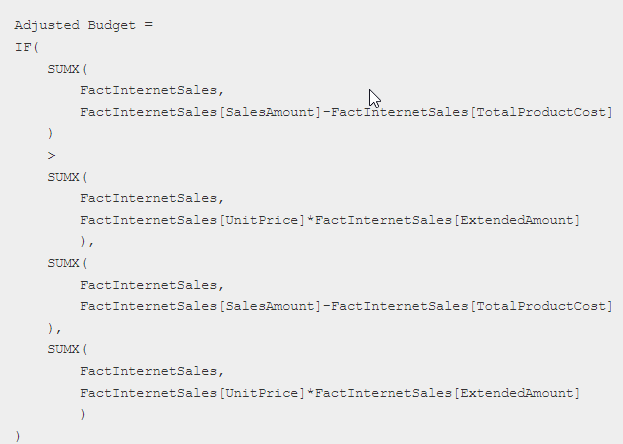The expression above is hard to read and also has some repetitive sections; let me mark them for you for better understanding:

We have two main parts in the expression above: A and B. Each of those is doing a calculation. Now, with the markings above, reading the expression is much simpler. The whole expression means this:

=IF(A>B, A, B)

All the above expression says is that if A is bigger than B, then return A; otherwise, B. It is much simpler to read because we split the repetitive parts into sections. That is what exactly the DAX variable is for.

## DAX Variables

You can define a DAX variable with VAR (not case-sensitive) and then re-use it as many times as you want through the same expression. Here is, for example, how I define a variable for A: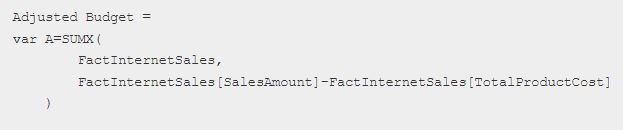Variables can be defined in any of the calculation objects: Column, Table, or Measure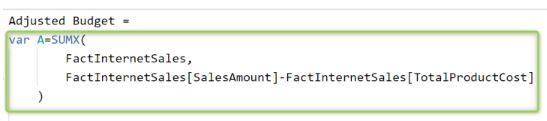The expression above is not yet a complete one, and if you try something like that, you will get an error. defining the variable is part of the operation. The other part is to return something. that is what we do using the RETURN keyword.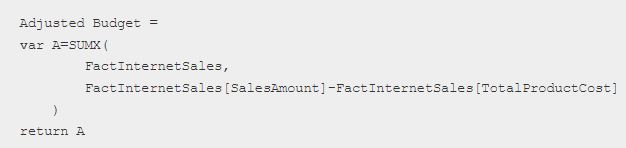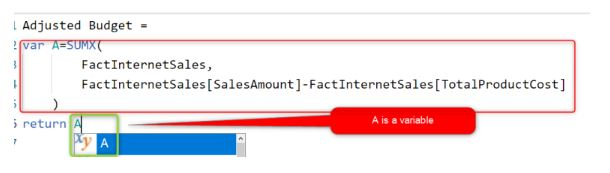This expression just defines a variable and returns it. We don’t really use the part of the expression defined within the variable more than once, but still ok.

You can define more variables by adding more VAR to the statement. Here is what our expression looks like using the variables: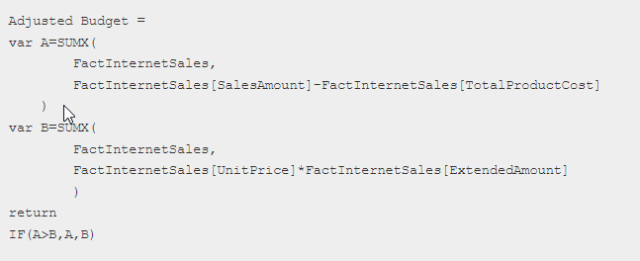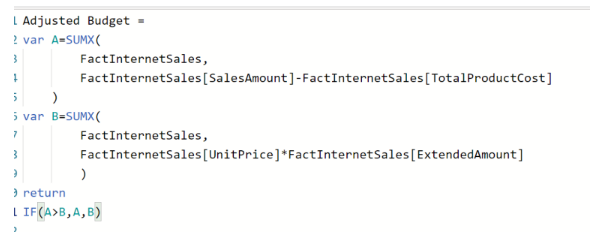## Variable Makes Your Code More Readable and More Consistent

The example above clearly shows how variables can make your code more readable. Instead of having an expression that is long and uses a lot of repetitive parts, you can define the repetitive part of the expression once and re-use it as many times as you want. when you define a part of the expression once and re-use it multiple times, your code will become more consistent too, and easier to maintain.

## Variables are Better for Performance

Variables are not just good for readability; they are also good for performance. In the expression below: the calculation for part A has been done twice, same for part B: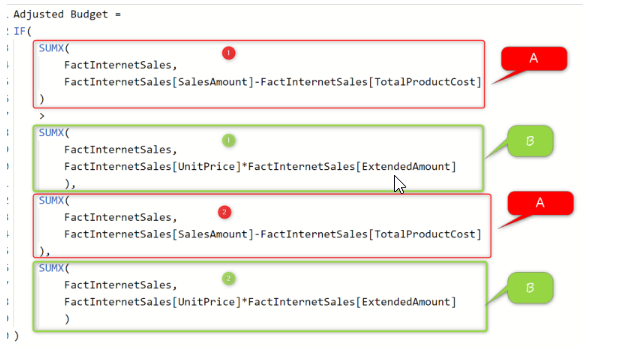However, if you define the part as a variable, then calculation happens once, and the result is stored in the variable and re-used multiple times. This would perform much faster than re-calculating it.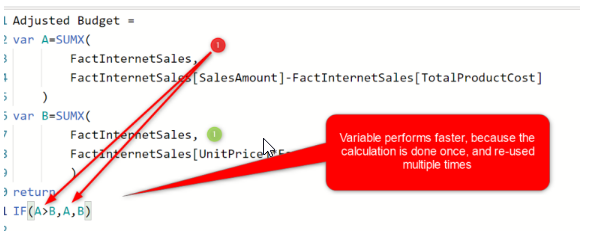## Anything can be Stored in a Variable: Table or Value

Another good thing about the variable is that you can even store a table in a variable. Like below: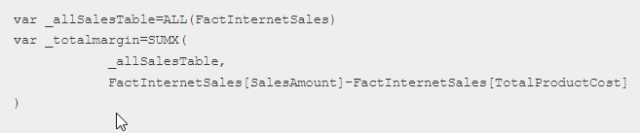As you can see, in this expression, ALL of the FactInternetSales are stored in a variable. This is a whole table stored in a variable and can be used in other places.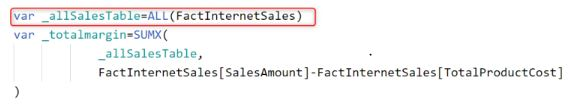## Variables can be Created Inline: Scope

a variable definition should not always be the start of your DAX code or even in the main part. it can be somewhere inline in another function. The expression below shows another way of writing for the last expression you have seen above.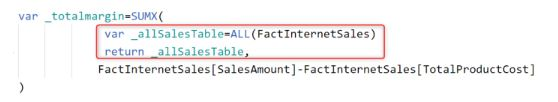## Variables vs. Measures

One of the questions you might have now is that; sometimes, for re-using an expression, you create a measure for it and then use that measure in the next expression. How is that different from a variable?

### Measures are Global, Variables are Local

Measures are global and can be used in any other measures in the same Power BI file. However, variables are local and can be used only in the expression in which they are defined.

### Variables are Better for Performance

Because of the nature of the way that variable is calculated, it would perform faster than using a measure instead of that. If you define a variable, the calculation for that variable is done once. However, if you create measures and then re-use measures multiple times in an expression, that measure calculation is done multiple times.

a workaround for this is to create a measure and then define a variable to materialize that measure in your expression. like in the below example, I have created a variable from the Sales measure: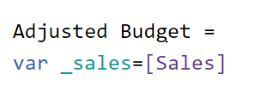## When Should You Create a Variable?

You might ask: OK, I understand what variable is, but I cannot understand a scenario to use it, or where in my DAX calculations should I change and add a variable? That is a very good question. Here are my rules of thumb for creating variables:

• If you use a part of your expression more than once
• If your expression is hard to read
• if the calculation is slow, and within that expression, you re-use part of the expression (note that this might be somewhere in the hierarchy of the measure, if you are pointing to a measure and that is using another measure, etc. somewhere down in the hierarchy, you might have used a section more than once)

## Summary

Variables, in my opinion, are the hidden gem of DAX expressions in Power BI and other tools that use DAX. Using variables, you will have a better code. Your code would be more readable, more consistent, easier to maintain, and most importantly, performs faster.

Happy Reading & Learning !!

## Thoughts on “Introduction to DAX Variables in Power BI”

1.Rakesh Gavel

Great ! Nice Blog !!

This site uses Akismet to reduce spam. Learn how your comment data is processed.

##### Arpit Malviya

Arpit Malviya has worked at Perficient as a technical consultant. He has been working in the Power BI & Data Analysis field since 2019.

More from this Author